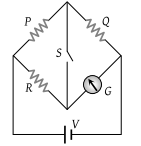# NEET Physics Current Electricity Questions Solved

In the circuit shown $P\ne R$, the reading of the galvanometer is same with switch S open or closed. Then(1) ${I}_{R}={I}_{G}$

(2) ${I}_{P}={I}_{G}$

(3) ${I}_{Q}={I}_{G}$

(4) ${I}_{Q}={I}_{R}$